The Normal Distribution

# 31 Using the Normal Distribution

The shaded area in the following graph indicates the area to the right of x. This area is represented by the probability P(X > x). Normal tables provide the probability between the mean, zero for the standard normal distribution, and a specific value such as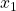. This is the unshaded part of the graph from the mean to.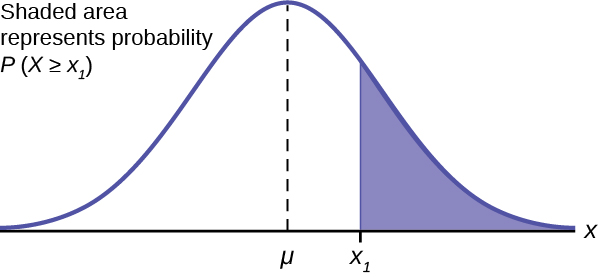Because the normal distribution is symmetrical , ifwere the same distance to the left of the mean the area, probability, in the left tail, would be the same as the shaded area in the right tail. Also, bear in mind that because of the symmetry of this distribution, one-half of the probability is to the right of the mean and one-half is to the left of the mean.

### Calculations of Probabilities

To find the probability for probability density functions with a continuous random variable we need to calculate the area under the function across the values of X we are interested in. For the normal distribution this seems a difficult task given the complexity of the formula. There is, however, a simply way to get what we want. Here again is the formula for the normal distribution: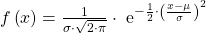Looking at the formula for the normal distribution it is not clear just how we are going to solve for the probability doing it the same way we did it with the previous probability functions. There we put the data into the formula and did the math.

To solve this puzzle we start knowing that the area under a probability density function is the probability.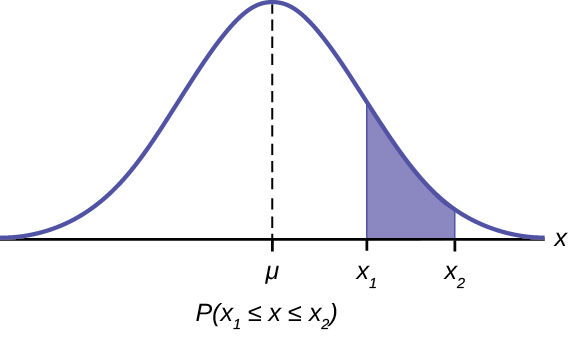This shows that the area between X1 and X2 is the probability as stated in the formula: P (X1 ≤ x ≤ X2)

The mathematical tool needed to find the area under a curve is integral calculus. The integral of the normal probability density function between the two points x1 and x2 is the area under the curve between these two points and is the probability between these two points.

Doing these integrals is no fun and can be very time consuming. But now, remembering that there are an infinite number of normal distributions out there, we can consider the one with a mean of zero and a standard deviation of 1. This particular normal distribution is given the name Standard Normal Distribution. Putting these values into the formula it reduces to a very simple equation. We can now quite easily calculate all probabilities for any value of x, for this particular normal distribution, that has a mean of zero and a standard deviation of 1. These have been produced and are available here in the appendix to the text or everywhere on the web. They are presented in various ways. The table in this text is the most common presentation and is set up with probabilities for one-half the distribution beginning with zero, the mean, and moving outward. The shaded area in the graph at the top of the table in Statistical Tables represents the probability from zero to the specific Z value noted on the horizontal axis, Z.

The only problem is that even with this table, it would be a ridiculous coincidence that our data had a mean of zero and a standard deviation of one. The solution is to convert the distribution we have with its mean and standard deviation to this new Standard Normal Distribution. The Standard Normal has a random variable called Z.

Using the standard normal table, typically called the normal table, to find the probability of one standard deviation, go to the Z column, reading down to 1.0 and then read at column 0. That number, 0.3413 is the probability from zero to 1 standard deviation. At the top of the table is the shaded area in the distribution which is the probability for one standard deviation. The table has solved our integral calculus problem. But only if our data has a mean of zero and a standard deviation of 1.

However, the essential point here is, the probability for one standard deviation on one normal distribution is the same on every normal distribution. If the population data set has a mean of 10 and a standard deviation of 5 then the probability from 10 to 15, one standard deviation, is the same as from zero to 1, one standard deviation on the standard normal distribution. To compute probabilities, areas, for any normal distribution, we need only to convert the particular normal distribution to the standard normal distribution and look up the answer in the tables. As review, here again is the standardizing formula: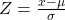where Z is the value on the standard normal distribution, X is the value from a normal distribution one wishes to convert to the standard normal, μ and σ are, respectively, the mean and standard deviation of that population. Note that the equation uses μ and σ which denotes population parameters. This is still dealing with probability so we always are dealing with the population, with known parameter values and a known distribution. It is also important to note that because the normal distribution is symmetrical it does not matter if the z-score is positive or negative when calculating a probability. One standard deviation to the left (negative Z-score) covers the same area as one standard deviation to the right (positive Z-score). This fact is why the Standard Normal tables do not provide areas for the left side of the distribution. Because of this symmetry, the Z-score formula is sometimes written as: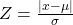Where the vertical lines in the equation means the absolute value of the number.

What the standardizing formula is really doing is computing the number of standard deviations X is from the mean of its own distribution. The standardizing formula and the concept of counting standard deviations from the mean is the secret of all that we will do in this statistics class. The reason this is true is that all of statistics boils down to variation, and the counting of standard deviations is a measure of variation.

This formula, in many disguises, will reappear over and over throughout this course.

The final exam scores in a statistics class were normally distributed with a mean of 63 and a standard deviation of five.

a. Find the probability that a randomly selected student scored more than 65 on the exam.
b. Find the probability that a randomly selected student scored less than 85.

a. Let X = a score on the final exam. X ~ N(63, 5), where μ = 63 and σ = 5.

Draw a graph.

Then, find P(x > 65).

P(x > 65) = 0.3446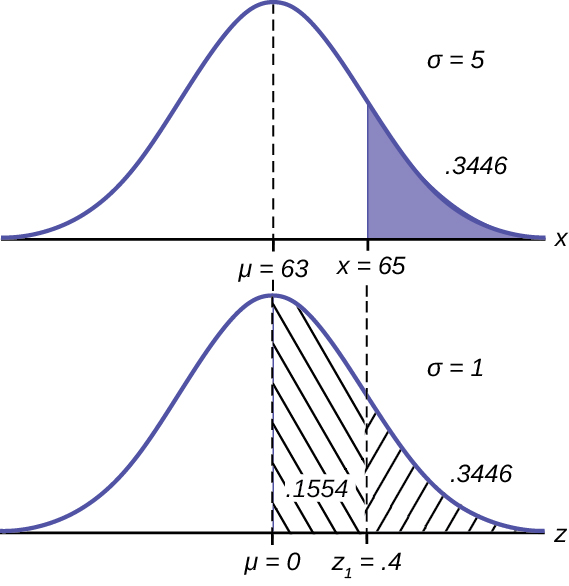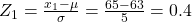P(xx1) = P(ZZ1) = 0.3446

The probability that any student selected at random scores more than 65 is 0.3446. Here is how we found this answer.

The normal table provides probabilities from zero to the value Z1. For this problem the question can be written as: P(X ≥ 65) = P(Z ≥ Z1), which is the area in the tail. To find this area the formula would be 0.5 – P(X ≤ 65). One half of the probability is above the mean value because this is a symmetrical distribution. The graph shows how to find the area in the tail by subtracting that portion from the mean, zero, to the Z1 value. The final answer is: P(X ≥ 63) = P(Z ≥ 0.4) = 0.3446

z =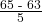= 0.4

Area to the left of Z1 to the mean of zero is 0.1554

P(x > 65) = P(z > 0.4) = 0.5 – 0.1554 = 0.3446

b.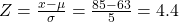which is larger than the maximum value on the Standard Normal Table. Therefore, the probability that one student scores less than 85 is approximately one or 100%.

A score of 85 is 4.4 standard deviations from the mean of 63 which is beyond the range of the standard normal table. Therefore, the probability that one student scores less than 85 is approximately one (or 100%).

Try It

The golf scores for a school team were normally distributed with a mean of 68 and a standard deviation of three.

Find the probability that a randomly selected golfer scored less than 65.

normalcdf(0,65,68,3) = 0.1587

A personal computer is used for office work at home, research, communication, personal finances, education, entertainment, social networking, and a myriad of other things. Suppose that the average number of hours a household personal computer is used for entertainment is two hours per day. Assume the times for entertainment are normally distributed and the standard deviation for the times is half an hour.

a. Find the probability that a household personal computer is used for entertainment between 1.8 and 2.75 hours per day.

a. Let X = the amount of time (in hours) a household personal computer is used for entertainment. X ~ N(2, 0.5) where μ = 2 and σ = 0.5.

Find P(1.8 < x < 2.75).

The probability for which you are looking is the area between x = 1.8 and x = 2.75. P(1.8 < x < 2.75) = 0.5886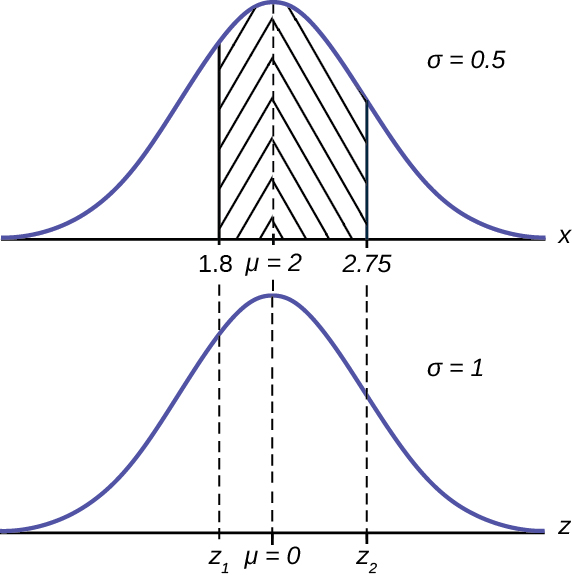P(1.8 ≤ x ≤ 2.75) = P(ZiZZ2)

The probability that a household personal computer is used between 1.8 and 2.75 hours per day for entertainment is 0.5886.

b. Find the maximum number of hours per day that the bottom quartile of households uses a personal computer for entertainment.

b. To find the maximum number of hours per day that the bottom quartile of households uses a personal computer for entertainment, find the 25th percentile, k, where P(x < k) = 0.25.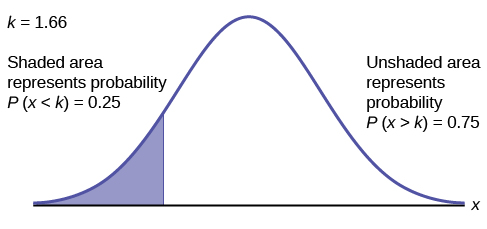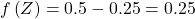, therefore Z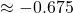(or just 0.67 using the table)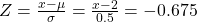, therefore x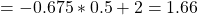hours.

The maximum number of hours per day that the bottom quartile of households uses a personal computer for entertainment is 1.66 hours.

Try It

The golf scores for a school team were normally distributed with a mean of 68 and a standard deviation of three. Find the probability that a golfer scored between 66 and 70.

normalcdf(66,70,68,3) = 0.4950

In the United States the ages 13 to 55+ of smartphone users approximately follow a normal distribution with approximate mean and standard deviation of 36.9 years and 13.9 years, respectively.

a. Determine the probability that a random smartphone user in the age range 13 to 55+ is between 23 and 64.7 years old.

a. 0.8186

b. Determine the probability that a randomly selected smartphone user in the age range 13 to 55+ is at most 50.8 years old.

b. 0.8413

A citrus farmer who grows mandarin oranges finds that the diameters of mandarin oranges harvested on his farm follow a normal distribution with a mean diameter of 5.85 cm and a standard deviation of 0.24 cm.

a. Find the probability that a randomly selected mandarin orange from this farm has a diameter larger than 6.0 cm. Sketch the graph.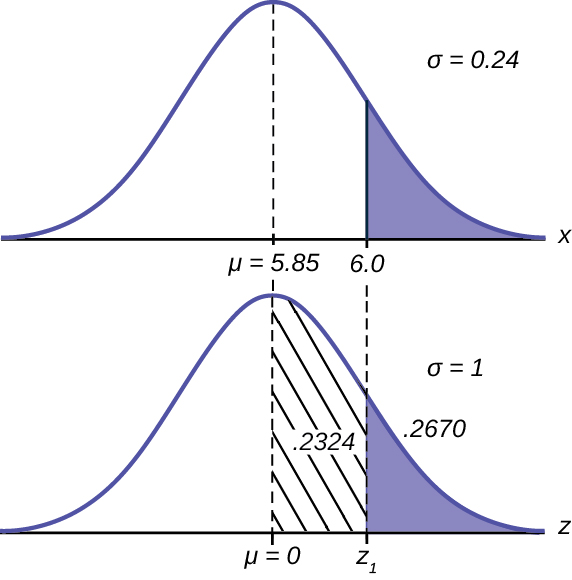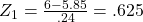P(x ≥ 6) = P(z ≥ 0.625) = 0.2670

b. The middle 20% of mandarin oranges from this farm have diameters between ______ and ______.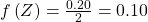, therefore Z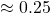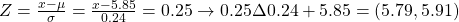### References

“Naegele’s rule.” Wikipedia. Available online at http://en.wikipedia.org/wiki/Naegele’s_rule (accessed May 14, 2013).

“403: NUMMI.” Chicago Public Media & Ira Glass, 2013. Available online at http://www.thisamericanlife.org/radio-archives/episode/403/nummi (accessed May 14, 2013).

“Scratch-Off Lottery Ticket Playing Tips.” WinAtTheLottery.com, 2013. Available online at http://www.winatthelottery.com/public/department40.cfm (accessed May 14, 2013).

“Smart Phone Users, By The Numbers.” Visual.ly, 2013. Available online at http://visual.ly/smart-phone-users-numbers (accessed May 14, 2013).